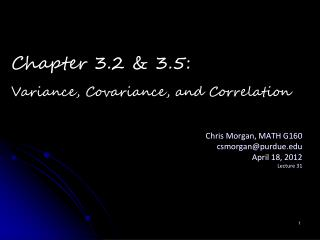Download PresentationChris Morgan, MATH G160 csmorgan@purdue.edu April 18, 2012 Lecture 31# Chris Morgan, MATH G160 csmorgan@purdue.edu April 18, 2012 Lecture 31 - PowerPoint PPT Presentation

Download Presentation##### Chris Morgan, MATH G160 csmorgan@purdue.edu April 18, 2012 Lecture 31

Download Policy: Content on the Website is provided to you AS IS for your information and personal use and may not be sold / licensed / shared on other websites without getting consent from its author. While downloading, if for some reason you are not able to download a presentation, the publisher may have deleted the file from their server.

- - - - - - - - - - - - - - - - - - - - - - - - - - - E N D - - - - - - - - - - - - - - - - - - - - - - - - - - -
##### Presentation Transcript

1. Chris Morgan, MATH G160 csmorgan@purdue.edu April 18, 2012 Lecture 31 Chapter 3.2 & 3.5: Variance, Covariance, and Correlation

2. Two different machines produce products with weights as follows: • Machine 1: 99 97 98 101 101 101 102 100 100 101 • Machine 2: 90 105 110 85 110 95 102 103 110 90 • mean of machine 1 = mean of machine 2 = 100 • Even though means are equivalent, very different outputs • Variance is the measure of difference between the value of each observation and the mean. Why do we care about Variability?

3. Example (I) Age of 40 students in the room today: 20 21 20 22 20 23 21 22 23 24 21 19 20 22 21 22 18 19 19 … Population (all 40 students): mean = 22.32 variance: 18 Sample of size 5: 20 21 20 23 21 mean = 21 variance: 1.5 Standard Deviation is the square root of variance and is denoted by sigma, σ. Population standard deviation: sqrt(18) = 4.24 Sample standard deviation: sqrt(1.5) = 1.22

4. Example (II) Number of wins by Minnesota Vikings last 10 seasons: 9 7 8 12 10 9 5 7 8 9 Population (all 10 seasons): mean = 8.4 variance: 40.2/10 = 4.02 Sample of size 4: 9 12 5 7 mean = 8.25 variance: 26.75/3 = 8.92

5. Coefficient of Variation • Coefficient of variation is the ratio of standard deviation to mean • Takes both location and variability into consideration • Reflect the relative variability of a population of sample

6. Covariance and Correlation Coefficient • • Measure the linear association between two variables, X and Y • • X is not necessarily a dependent variable • • The population size or sample size of X and Y must equal • Correlation Coefficient represented by r

7. Example (III)

8. Example (III) • X: = 8.375, s2X = 17.98, sx = 4.24 • Y: = 18.31, s2Y =193.43, sy = 13.91 • sXY = 52.54 • r = sxy/(sx*sy) = 52.54 / (4.24*13.91) = 0.89

9. Example (IV) You give out a satisfaction survey to your employees (Executives and Middle Managers) and they rate their satisfaction on a scale of 1 to 5, 1 being least satisfied and 5 being most satisfied. You find the following: Executives: Mean score = 4.0 Standard Deviation = 1.7 Middle Managers: Mean score = 3.5 Standard Deviation = .8 • What does this tell you about your employees? • Using this data, what would you want to do to try to improve your employee satisfaction? How would it be different for Middle Managers and Executives? Why?

10. Example (V) In this company that you’re running, you decide to have a supplier ship office supplies every month to keep your inventory up and your employees happy. You look into a couple different suppliers of their time to ship: • Which supplier would you go with and why? • What other factors would you want to see before you make your decision?

11. And now for the supplement…